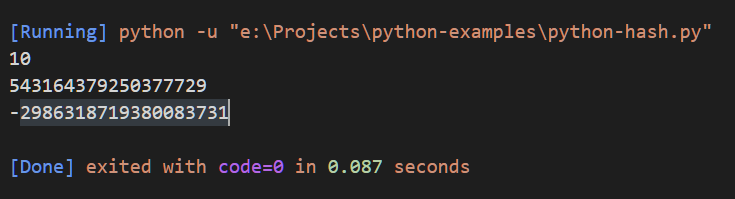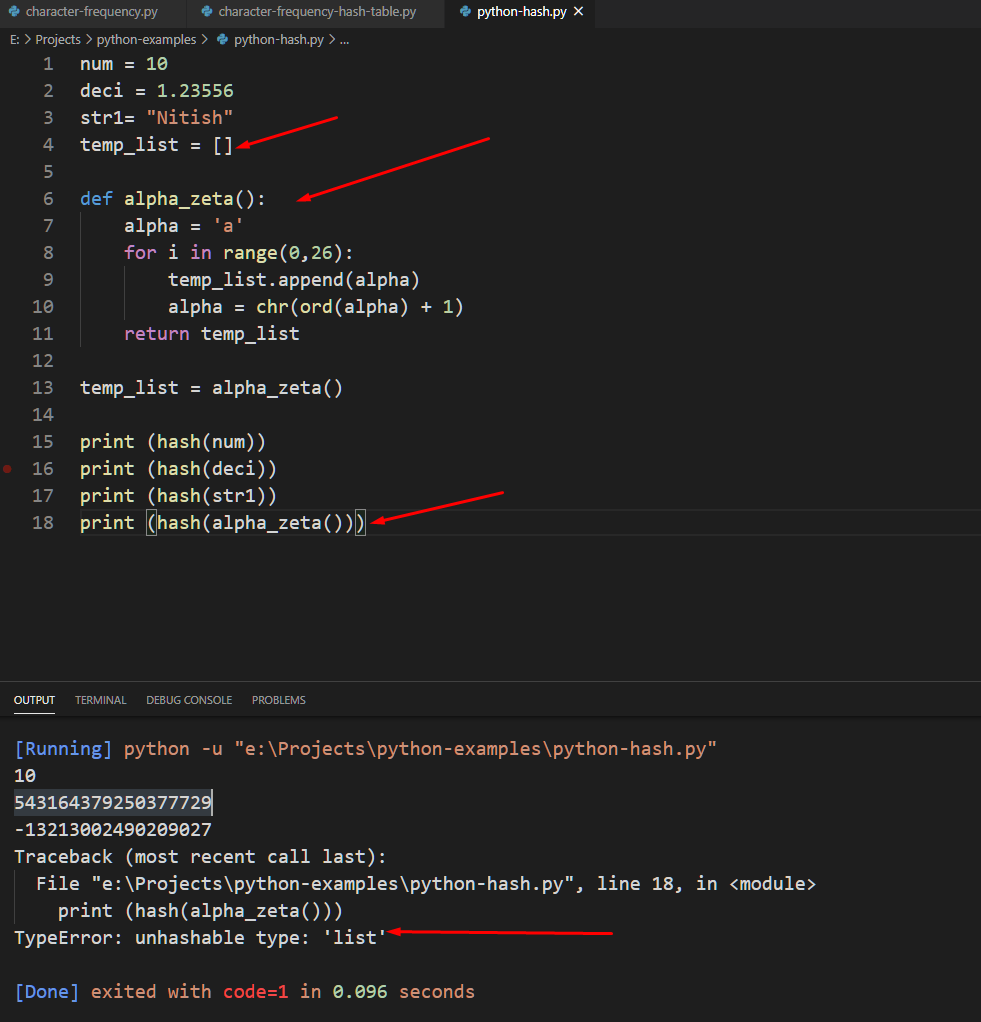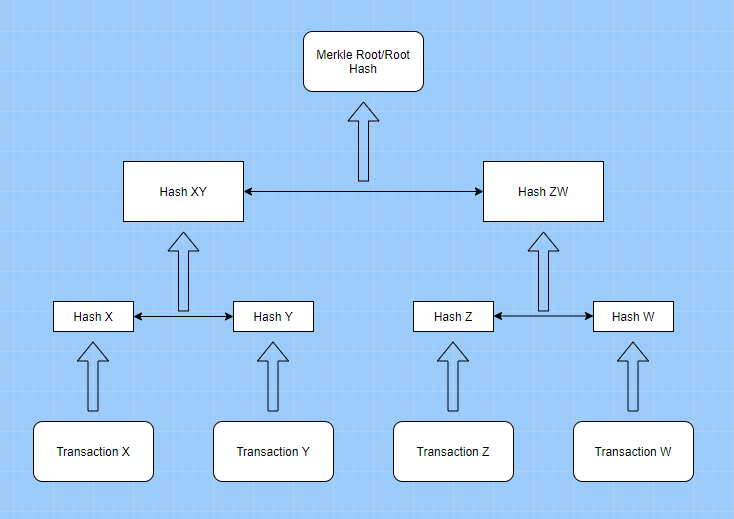Are you looking to learn about hashing in cryptography? If you do, then you have come to the right place. In this article, we will explore more about hashing. Hashing is a computer science technique for identifying objects or values from a group of objects or values. Sounds confusing? Let’s try to understand by example.

Well, colleges and schools provide a uniquely assigned number to each of their students. This unique number is what identifies a student and the information related to him. The method used to generate the unique number is Hashing.

Another popular example is libraries where you will find tons of books on the shelves. Each book there has its unique identification number so that it can be located in the huge library!

A modern example of hashing would be game players that register for the game. Valorant is a free-to-play game launched by Riot. Being free-to-play means that millions of people will play the game.

Build your identity as a certified blockchain expert with 101 Blockchains’ Blockchain Certifications designed to provide enhanced career prospects.

Each player is identified using a unique identification value generated using a hashing algorithm.```Please include attribution to 101blockchains.com with this graphic. <a href='https://101blockchains.com/blockchain-infographics/'> <img src='https://101blockchains.com/wp-content/uploads/2020/07/Hashing_in_cryptography.png' alt='Hashing in cryptography='0' /> </a>
```

Let’s try to understand it in more detail below

## What is Hashing?

As stated above, Hashing is the method of identifying an object from a group. Each object gets a unique identification number once hashed. But, what does that mean technically? Technically, a mathematical function generates a fixed-length output from any input string of any length.

Bitcoin transactions are hashed where the transactions get unique IDs. If you put in “Hello, World!” in an SHA-256 hashing algorithm, you will get the following output:

Input: Hello, World!

Output: dffd6021bb2bd5b0af676290809ec3a53191dd81c7f70a4b28688a362182986f

Here SHA256 generates the output from the given input. As you can see, we used the Secure Hash Function(SHA-256) hashing algorithm. It is one of the popular hashing methods out there, including Message Direct(MD5), and Secure Hash Function(SHA1).

The key properties of the hash function make it reliable. Let’s list them below.

• Deterministic → This means that the output will be the same for the given input in any given circumstances.
• Preimage Resistant → The preimage resistant feature ensures that the hash value is not useful for generating the input value.
• Computationally efficient → The hash functions are efficient and don’t require huge computational resources to execute.
• Cannot be reversed engineered → The hash function cannot be reversed engineered.
• Collision resistant → Collision resistance ensures that no two inputs result in the same output.

But, if you are here for advanced stuff, you will not be disappointed.

Want to become a Cryptocurrency expert? Enroll Now in Cryptocurrency Fundamentals Course

### What is Hash Function And Hash Tables? And How Do They Work?

In this section, we will explore the hash function and hash tables in more detail. In terms of hashing, there are hash functions. These functions are responsible for converting large inputs into small fixed inputs. The hash tables store the outputs.

In the hashing process, the objects are distributed based on their key/value pairs to the array. So, if you pass an array of elements to the hash functions, you will get an array output where each of the elements now has a key attached to it. The key/value pair is very useful when it comes to accessing elements in real-time as it offers an impressive O(1) time.

To implement hash functions, you can take off the two preferred approaches.

• The first approach is to use a hash function to convert an element to an integer. Next, the integer output can be used to access the element when putting in the hash table.
• Another step is to put the element in the hash table and then retrieve it using the hashed key.

In the 2nd method, the functions will be as below:

```hash = hash_function(key)

index = hash % array_size```

Here, the hash and the array sizes are independent of each other. The index value is calculated based on the array size. Modulo operator(%) enables us to calculate the value.

In simple terms, a hash function can be defined as a function that can map arbitrary size data set to a fixed size data set. The resulting fixed sized data set can be stored in the hash table. Many names are given to the values returned by the hash function. They can be called hash values, hashes, hash sums, and hash codes.

• #### Writing A Good Hash Function

If you want to create a good hash function or mechanism, you need to understand the basic requirements of creating one. Let’s list them below:

• The hash function needs to be easy to compute. That means that it shouldn’t take many resources to execute.
• The hash function needs to be uniformly distributed. By doing so, hash tables are utilized for storing the hash values so that clustering won’t happen.
• The last requirement is to have less or no collision at all. No collision means that no single output is mapped to two inputs.

Technically, collisions are part of a hash function, and it simply cannot be removed from a hash function. The goal is to create a hash function that can offer good hash table performance and resolve collision through collision resolution techniques.

Start learning about Cryptocurrencies with World’s first Cryptocurrency Skill Path with quality resources tailored by industry experts Now!

• #### Why Do We Need A Good Hash Function?

To understand the need for a useful hash function, let’s go through an example below. Let’s assume that we want to create a Hash table using a hashing technique where the input strings will be as follows, {“agk”, “kag”, “gak”, “akg”, “kga”, “gka” }

Now, we create a hash function that simply adds the ASCII value of a(97), g(103), and k(107) and then does a modulo of the sum by 307.

Clearly, the sum of the three numbers is also 307. This means that if we permute all the numbers and then do a modulo operation, we will get the same result. The end result would be storing all the strings to the same index number. The algorithmic time for the hash function would also be O(n) complexity, which is not desirable. We can easily conclude that the hash function that we described is not optimal for real-life scenarios.

To fix the hash function, we can deploy dividing the sum of ASCII values of each element by another prime number, 727. By doing so, we will get a different output for our given input strings array.

• #### Learning About Hash Tables

The hash tables are very useful in storing the result of a hash function, which computes the index and then stores a value against it. The end result would be faster computational process with O(1) complexity.

Hash tables are traditionally a good choice in solving problems that require O(n) time.

So, if you pick up a string of a fixed length and then try to learn the string’s character frequency.

So, if string = “aacddce”,  then a generic approach is to go through the string multiple times and store each frequency.

#Provide an input string, and count the frequency of characters in that string

#The algorithm is 0(n) complexity time

```temp_list = []

start = "a"

str = "ababcddefff"

def alpha_zeta():

alpha = 'a'

for i in range(0,26):

temp_list.append(alpha)

alpha = chr(ord(alpha) + 1)

return temp_list

temp_list = alpha_zeta()

#print (temp_list)

def character_frequency(str, temp_list):

for each in temp_list:

freq = 0

for i in str:

if (i == each):

freq = freq + 1

print (each, freq)

character_frequency(str,temp_list)```

The output of the above program will be as follows:

```a 2

b 2

c 1

d 2

e 1

f 3

g 0

h 0

i 0

..

..

```

Now, let’s implement a hash table in C++ and count the character frequency.

```#include <iostream>

using namespace std;

int Frequency;

int hashFunc(char c) {

return (c - 'a');

}

void countFre(string S)

{

for (int i = 0; i< S.length(); ++i){

int index = hashFunc(S[i]);

Frequency[index]++;

}

for (int i = 0; i<26; ++i) {

cout << (char)(i+'a') << ' ' << Frequency[i] << endl;

}

}

int main()

{

cout<<"Hello World";

countFre("abbaccbdd");

}```

The output of the program would be as below:

```a 2

b 3

c 2

d 2```

The O(N) complexity of the algorithm makes it faster compared to other linear approaches.

• #### How to resolve Collisions

There are unique ways to solve collisions in hash functions. One of the popular ways is separate chaining which is also known as open hashing. It is implemented with a linked list where each of the elements in the chain is itself a linked list. This approach enables to store elements and ensure that certain elements are only part of the specific linked list, resolving collision. That means that no two input values can have the same output hash value.

### Exploring Hash in Python

In this section, we will quickly look out at hash in Python. The reason we choose Python is that it is easy to read and can be used by anyone without much trouble.

As hashing is a common function, it is already implemented in the Python library. By using the module, you can provide an object as its input and then return the hashed value.

The syntax of the hash method is:

hash(object)

As you can see, it takes in a single parameter, which is the object. The object can be integer, float, or string.

The returned value of the hash() method depends on the input. For an integer, it might return the same number whereas for decimal and string would be different.

Let’s see some examples below.

```num = 10

deci = 1.23556

str1= "Nitish"```
```print (hash(num))

print (hash(deci))

print (hash(str1))```

The output of the above code is as below:However, hashing cannot be applied to all object types. For example, if you remember that we created a list of a to z in our first program. If we try to hash it, the output window will through a TypeError: unhashable type: ‘list’To apply hashing to an object list, you need to use tuple.

```vowels = ('a', 'e','i','o','u')

print (hash(vowels))

Output ⇒ -5678652950122127926```

### Hashing in Cryptography

Hashing is useful to cryptography. Bitcoin utilizes hashing to create and manage Merkle trees Also, hashing has been part of cryptography for quite a long time. However, the best use case of hashing is to hash passwords and store them.

• #### Merkle Trees

Merkle tree is a data structure that is useful when it comes to doing secure data verification in a large data pool. Both Bitcoin and Ethereum utilize Merkle trees to solve many technological barriers when storing and accessing data in an open network.

Want to learn the basic and advanced concepts of Ethereum? Enroll Now: Ethereum Development Fundamentals

Any centralized network does not have to worry about storing and accessing data as there is only one source for both accessing and storing the data. However, the equation changes when there is a decentralized network as now the data needs to be copied among hundreds of participating peers.

Merkle trees solve the problem by providing a trusted and efficient way to share and verify data among peers.Merkle Tree Example

But, why are we discussing Merkle trees here? Merkle trees use the hash as the core functionality to connect the different nodes and data blocks.

Merkle Trees is an upside-down tree that can summarize the whole transaction set.

If you want to learn more about Merkle trees and how it uses hashing in cryptography, check out our detailed guide: A Guide To Merkle Trees. There, we discussed how Merkle trees implements are done in bitcoin and other use-cases.

• #### Mining Process

The mining process also takes advantage of hashing. When it comes to bitcoin mining, a new block is added to the blockchain when there is a demand for it. A  method needs to be followed to add the block to the blockchain. A hash value is generated depending on the block’s content when a new block arrives. Also, if the generated hash is more than network difficulty, the process of adding the block to blockchain gets started.

Once done, all the peers in the network acknowledge the addition of the new block. But, that is rarely the case as the network difficulty, in most cases, is always higher compared to the generated hash. There is another aspect that plays a crucial role in the mining process. It is the nonce.

The nonce is added to the block’s hash and is an arbitrary string. Once done, the concatenated string is then compared to the difficulty level. If the difficulty level is lower than the concatenated string, then the nonce is changed until the difficulty level is higher.

The process can be summarized in the following steps:

• The contents are hashed to create a new hash value whenever a new block is generated or taken,
• A new nonce value gets generated and appended to the hash
• Hashing process takes place on the new contacted string
• The final value of the hash is then compared with the network’s difficulty level
•  if the final hash value is lower than the nonce, the process is repeated again. The process only stops when the hash value is more than the nonce.
• Block joins the chain once the difficulty level is higher
• The miners then take the responsibility to mine the new block and share the rewards among themselves.

The term “hash rate” also comes in from here.  The Hash rate is the rate at which the hashing operations take place. A higher hash rate means that the miners would require more computation power to participate in the mining process.

Curious to learn about blockchain implementation and strategy for managing your blockchain projects? Enroll Now in Blockchain Technology – Implementation And Strategy Course!

### Conclusion

This leads us to the end of our hashing in cryptography in-depth guide. We covered hashing in detail and also explored the code behind it.*Disclaimer: The article should not be taken as, and is not intended to provide any investment advice. Claims made in this article do not constitute investment advice and should not be taken as such. 101 Blockchains shall not be responsible for any loss sustained by any person who relies on this article. Do your own research!